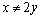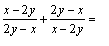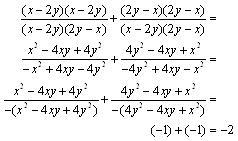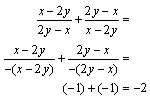# Extra Hard SAT Math Question – FOIL or Factor?SAT test writers are always trying to find new ways to discombobulate students.

At the end of every SAT Math section, the test makers try to come up with an extremely difficult problem that will leave even the cleverest students scratching their heads. The really evil part, though, is that even these problems can be solved in under a minute without a calculator – if you know what to do. This means that once you “figure out the trick,” these difficult problems become easy. So, while those test makers are busy cackling with sadistic glee, let’s see if we can’t beat them at their own game.

Consider the following problem:

For all x and y where,A) 2(x – 2y)

B) 2y – x

C) 1

D) 0

E) -2

Well, as you know, you can’t add fractions unless their denominators are the same, so if you want to add these two fractions, then you would have to multiply the numerator and denominator of the first fraction by the denominator of the second fraction and the numerator and denominator of the second fraction by the denominator of the first fraction, like so:So, the answer is E. But that’s the long way. By the time you get to the third line of all that awful math, you should realize that the numerator and denominator of each fraction are essentially the same, except that one has been multiplied by -1. In line 3 above, we factor out the -1 and realize that these fractions are just a glorified way of writing -1 + -1, but if we had stopped to think about it at the beginning, we could have realized it without multiplying all those binomials:Granted, if you remember the FOIL method, multiplying the binomials shouldn’t take that long, but it’s still nice if you can do a 2 minute problem in 10 seconds, since that gives you more time for other questions you may need to think about. A method with fewer steps also leaves less room for careless errors. But how are you going to find these shortcuts on the day of the test? Ironically, if you are rushing through the test as fast as you can, you’re more likely to do problems the long way and miss the shortcuts. Before you dive into a problem and break out your calculator, pause and reflect: every SAT math problem can be solved in under a minute without a calculator. This means that for the harder problems, there is always some trick. If you ever find yourself scribbling down lines and lines of scratch work, that probably means that you’re doing a problem the long way. For this problem, just look at it. You will notice a lot of repetition: everything is made up of x and 2y. This is a red flag that there’s a trick to solving this problem. If everything is kind of the same, then if you arrange the expressions properly chances are things will start to cancel out. In this case, you have to factor out a -1 from either the numerators or the denominators. After that, it becomes clear that each fraction is equal to -1, and the problem becomes, as they say, easy as π!

READ  SAT vs. ACT: Which Test to Take and Score Conversion

So you see, with patience and practice, even the hardest problems on the SAT become easy. As you do more practice problems you will get better and better at spotting these shortcuts – the test makers tend to use the same tricks over and over again. Check back here each week for more extra hard problems and the tricks you need to solve them! Also, remember that you can find out all the tricks from experts like me with a Test Masters course or private tutoring. Until  then, keep up the good work and happy studying!

### You Might Also Like

1.Nick says:

I don’t understand why do you leave the (-) sign in front of the (x – 2y) and the (2y – x) after they have already been multiplied by (-1), because that would mean that:
-(x – 2y) would be equal to -x + 2y, and that -(2y – x) would be equal to
-2y + x. Can you please explain this. Thank you in advance.

1.Bill says:

Nick,

We’re not sure we understand your question. -(x-2y) IS equal -x + 2y, and (-2y – x) is equal to -2y +x. Also, you’re not actually multiplying the expressions by -1, you’re just factoring the -1 in the denominator. Doing that makes it more obvious that the expression has a value of -1.

Hope this helps!